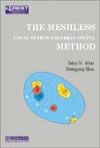The Meshless Local Petrov-Galerkin (MLPG) Method

The Meshless Local Petrov-Galerkin (MLPG) Method

Edited by S. N.Atluri, S. Shen

This book is the first monograph (in the new series, CREST), on this new class of meshless methods, that are expected to revolutionalize engineering/science analyses. It not only deals with several meshless methods in general, but also with the Meshhless Local Petrov-Galerkin (MLPG) method, pioneered by the authors, in particular.Chapter Titles:

Chapter 1.

Global weak form and the weighted residual method (WRM); Point collocation method; Weighted integral square error; Subdomain integral/average error method; Finite volume method; Finite element method; Boundary element Method; Local weak forms;

Chapter 2.

Meshless interpolation of trial functions; Moving least-square approximation; Generalized moving least-square approximation; The influences of the weight function; Shepard functions;Partition of unity methods; Reproduced kernel particle method; Radial basis functions; Interpolation errors in meshless interpolations;

Chapter 3.

Meshless local Petrov-Galerkin method(MLPG); Numerical implementation of the MLPG; Six different Types of MLPG methods; Essential boundary conditions; Efficient Meshless Numerical integration; Numerical examples;Patch test,Laplace equation,Possion's equation; An analysis of the Computational costs; The nonlinear problem;

Chapter 4.

Application of MLPG in elasticity; The linear elasto-static problem;Arbitrary placement of secondary nodes, and error control; Elastostatic fracture; Analysis of beams using GMLS; Analysis of Shear-Flexible Beams & Shells;

Chapter 5.

Application of MLPG in fluid mechanics; The convection-diffusion problems; Berger's equations; Incompressible Navier-Stokes equations;

Chapter 6.

Prospects for future research: Nonlinear Analysis of Shells; Gradient Theories of inelasticity; Strain-localization; Nanotechnoloty.

Hardcover. 440 pages. © 2002. ISBN: 0-9657001-5-1.